## Free Statistics

of Irreproducible Research!

Author's title

# Bootstrap Plot (gemiddelde consumptieprijzen aardappelen/kg, 2006-2011), 20...

Author*Unverified author*
R Software Modulerwasp_bootstrapplot.wasp
Title produced by softwareBlocked Bootstrap Plot - Central Tendency
Date of computationThu, 27 Dec 2012 15:42:04 -0500
Cite this page as followsStatistical Computations at FreeStatistics.org, Office for Research Development and Education, URL https://freestatistics.org/blog/index.php?v=date/2012/Dec/27/t13566409427g594244hc5glys.htm/, Retrieved Tue, 31 Jan 2023 07:48:58 +0000
Statistical Computations at FreeStatistics.org, Office for Research Development and Education, URL https://freestatistics.org/blog/index.php?pk=204789, Retrieved Tue, 31 Jan 2023 07:48:58 +0000
QR Codes:Original text written by user:
IsPrivate?No (this computation is public)
User-defined keywords
Estimated Impact96
Family? (F = Feedback message, R = changed R code, M = changed R Module, P = changed Parameters, D = changed Data)
-     [(Partial) Autocorrelation Function] [Autocorrelatie In...] [2012-11-12 10:59:56] [41982c7b3984978a38ca838fef047984]
- RMPD  [Bootstrap Plot - Central Tendency] [Bootstrap Plot (m...] [2012-12-27 20:12:14] [41982c7b3984978a38ca838fef047984]
- R P     [Bootstrap Plot - Central Tendency] [Bootstrap Plot (m...] [2012-12-27 20:14:03] [41982c7b3984978a38ca838fef047984]
- RMPD      [Blocked Bootstrap Plot - Central Tendency] [Bootstrap Plot (g...] [2012-12-27 20:38:33] [41982c7b3984978a38ca838fef047984]
- R  D          [Blocked Bootstrap Plot - Central Tendency] [Bootstrap Plot (g...] [2012-12-27 20:42:04] [97ff841fcf87514e420f2e9629cfd808] [Current]
-                 [Blocked Bootstrap Plot - Central Tendency] [Bootstrap Plot (g...] [2012-12-27 20:44:01] [41982c7b3984978a38ca838fef047984]
- RM D            [Variability] [Spreidingsmaten S...] [2012-12-27 20:59:10] [41982c7b3984978a38ca838fef047984]
- RM D            [Standard Deviation Plot] [Spreidingsgrafiek...] [2012-12-27 21:03:45] [41982c7b3984978a38ca838fef047984]
- RM D            [Standard Deviation-Mean Plot] [Spreidings- en ge...] [2012-12-27 21:11:16] [41982c7b3984978a38ca838fef047984]
- RM D            [Variability] [Spreidingsmaten g...] [2012-12-27 21:17:49] [41982c7b3984978a38ca838fef047984]
- RM              [Standard Deviation Plot] [Spreidingsgrafiek...] [2012-12-27 21:20:17] [41982c7b3984978a38ca838fef047984]
- RM              [Standard Deviation-Mean Plot] [Spreidings- en ge...] [2012-12-27 21:24:21] [41982c7b3984978a38ca838fef047984]
- RM D            [Classical Decomposition] [Decompositie Insc...] [2012-12-27 21:30:41] [41982c7b3984978a38ca838fef047984]
Feedback Forum

Post a new message
Dataseries X:
0.75
0.75
0.77
0.78
0.79
1.01
1.16
1.14
1.12
1.1
1.1
1.1
1.1
1.09
1.09
1.1
1.1
1.17
1.15
1.04
0.94
0.88
0.85
0.85
0.85
0.84
0.83
0.8
0.78
1.02
1.19
1.1
0.96
0.87
0.83
0.82
0.81
0.78
0.79
0.8
0.79
0.97
1.01
0.92
0.87
0.84
0.81
0.81
0.83
0.83
0.85
0.88
0.89
1.21
1.32
1.33
1.23
1.16
1.12
1.06
1.08
1.09
1.03
1.04
1.05
1.19
1.14
1.05
0.95
0.87
0.86
0.85

 Summary of computational transaction Raw Input view raw input (R code) Raw Output view raw output of R engine Computing time 6 seconds R Server 'Sir Ronald Aylmer Fisher' @ fisher.wessa.net

\begin{tabular}{lllllllll}
\hline
Summary of computational transaction \tabularnewline
Raw Input & view raw input (R code)  \tabularnewline
Raw Output & view raw output of R engine  \tabularnewline
Computing time & 6 seconds \tabularnewline
R Server & 'Sir Ronald Aylmer Fisher' @ fisher.wessa.net \tabularnewline
\hline
\end{tabular}
%Source: https://freestatistics.org/blog/index.php?pk=204789&T=0

[TABLE]
[ROW][C]Summary of computational transaction[/C][/ROW]
[ROW][C]Raw Input[/C][C]view raw input (R code) [/C][/ROW]
[ROW][C]Raw Output[/C][C]view raw output of R engine [/C][/ROW]
[ROW][C]Computing time[/C][C]6 seconds[/C][/ROW]
[ROW][C]R Server[/C][C]'Sir Ronald Aylmer Fisher' @ fisher.wessa.net[/C][/ROW]
[/TABLE]
Source: https://freestatistics.org/blog/index.php?pk=204789&T=0

Globally Unique Identifier (entire table): ba.freestatistics.org/blog/index.php?pk=204789&T=0

As an alternative you can also use a QR Code:

The GUIDs for individual cells are displayed in the table below:

 Summary of computational transaction Raw Input view raw input (R code) Raw Output view raw output of R engine Computing time 6 seconds R Server 'Sir Ronald Aylmer Fisher' @ fisher.wessa.net

 Estimation Results of Blocked Bootstrap statistic Q1 Estimate Q3 S.D. IQR mean 0.950069444444445 0.97125 0.993923611111111 0.0358620310370244 0.0438541666666666 median 0.88375 0.955 1.035 0.0778583908137107 0.15125 midrange 0.985 1.04 1.04 0.0319849898055998 0.055

\begin{tabular}{lllllllll}
\hline
Estimation Results of Blocked Bootstrap \tabularnewline
statistic & Q1 & Estimate & Q3 & S.D. & IQR \tabularnewline
mean & 0.950069444444445 & 0.97125 & 0.993923611111111 & 0.0358620310370244 & 0.0438541666666666 \tabularnewline
median & 0.88375 & 0.955 & 1.035 & 0.0778583908137107 & 0.15125 \tabularnewline
midrange & 0.985 & 1.04 & 1.04 & 0.0319849898055998 & 0.055 \tabularnewline
\hline
\end{tabular}
%Source: https://freestatistics.org/blog/index.php?pk=204789&T=1

[TABLE]
[ROW][C]Estimation Results of Blocked Bootstrap[/C][/ROW]
[ROW][C]statistic[/C][C]Q1[/C][C]Estimate[/C][C]Q3[/C][C]S.D.[/C][C]IQR[/C][/ROW]
[ROW][C]mean[/C][C]0.950069444444445[/C][C]0.97125[/C][C]0.993923611111111[/C][C]0.0358620310370244[/C][C]0.0438541666666666[/C][/ROW]
[ROW][C]median[/C][C]0.88375[/C][C]0.955[/C][C]1.035[/C][C]0.0778583908137107[/C][C]0.15125[/C][/ROW]
[ROW][C]midrange[/C][C]0.985[/C][C]1.04[/C][C]1.04[/C][C]0.0319849898055998[/C][C]0.055[/C][/ROW]
[/TABLE]
Source: https://freestatistics.org/blog/index.php?pk=204789&T=1

Globally Unique Identifier (entire table): ba.freestatistics.org/blog/index.php?pk=204789&T=1

As an alternative you can also use a QR Code:

The GUIDs for individual cells are displayed in the table below:

 Estimation Results of Blocked Bootstrap statistic Q1 Estimate Q3 S.D. IQR mean 0.950069444444445 0.97125 0.993923611111111 0.0358620310370244 0.0438541666666666 median 0.88375 0.955 1.035 0.0778583908137107 0.15125 midrange 0.985 1.04 1.04 0.0319849898055998 0.055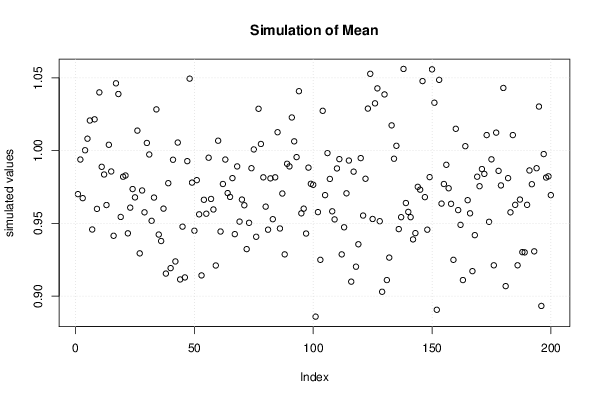PNG link Postscript link PDF link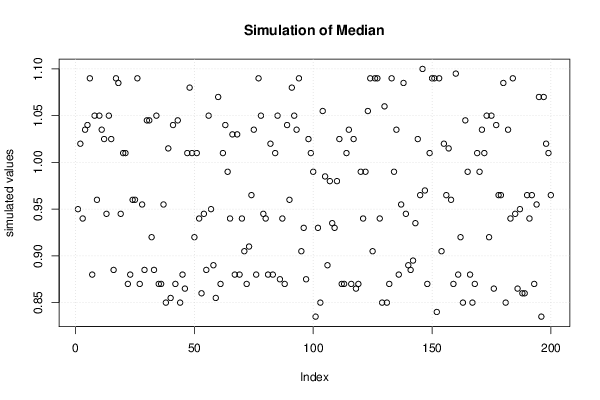PNG link Postscript link PDF link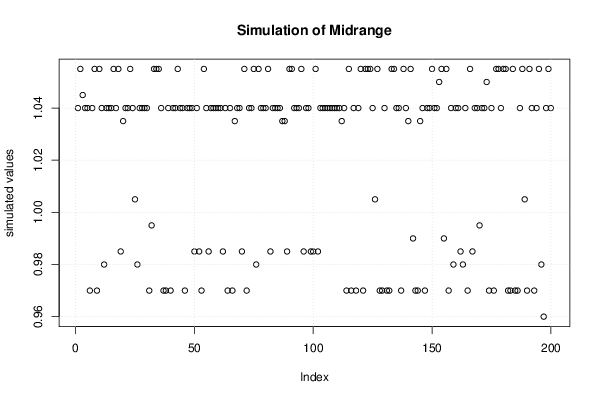PNG link Postscript link PDF link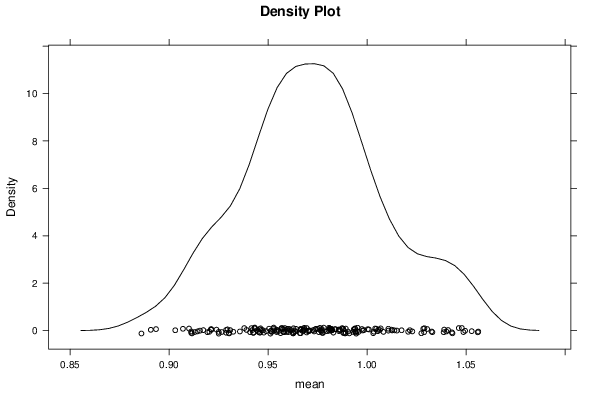PNG link Postscript link PDF link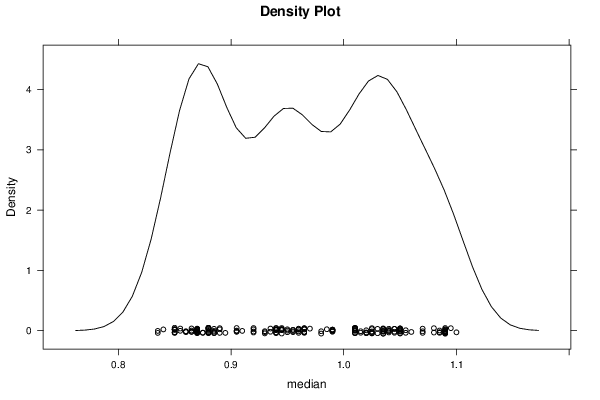PNG link Postscript link PDF link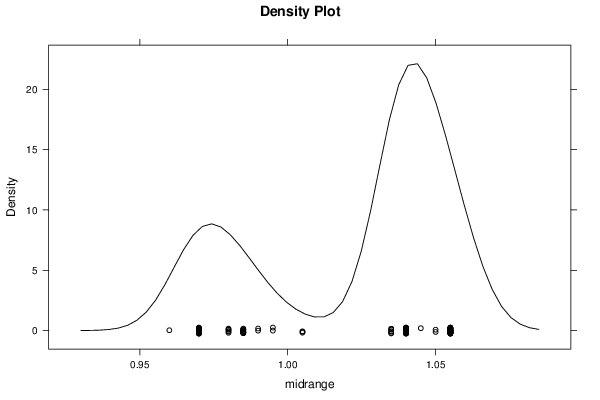PNG link Postscript link PDF link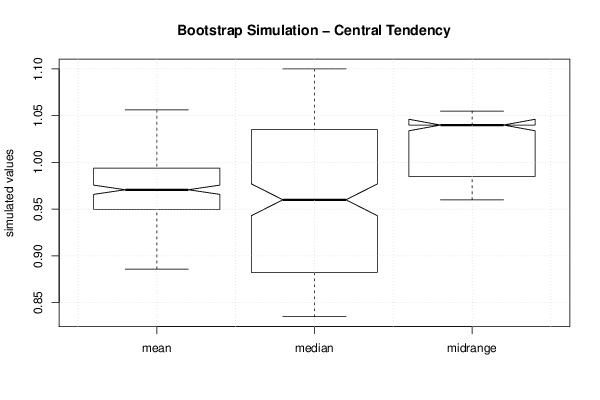PNG link Postscript link PDF link

Parameters (Session):
par1 = 50 ; par2 = 12 ;
Parameters (R input):
par1 = 200 ; par2 = 12 ;
R code (references can be found in the software module):
par1 <- as.numeric(par1)par2 <- as.numeric(par2)if (par1 < 10) par1 = 10if (par1 > 5000) par1 = 5000if (par2 < 3) par2 = 3if (par2 > length(x)) par2 = length(x)library(lattice)library(boot)boot.stat <- function(s){s.mean <- mean(s)s.median <- median(s)s.midrange <- (max(s) + min(s)) / 2c(s.mean, s.median, s.midrange)}(r <- tsboot(x, boot.stat, R=par1, l=12, sim='fixed'))bitmap(file='plot1.png')plot(r$t[,1],type='p',ylab='simulated values',main='Simulation of Mean')grid()dev.off()bitmap(file='plot2.png')plot(r$t[,2],type='p',ylab='simulated values',main='Simulation of Median')grid()dev.off()bitmap(file='plot3.png')plot(r$t[,3],type='p',ylab='simulated values',main='Simulation of Midrange')grid()dev.off()bitmap(file='plot4.png')densityplot(~r$t[,1],col='black',main='Density Plot',xlab='mean')dev.off()bitmap(file='plot5.png')densityplot(~r$t[,2],col='black',main='Density Plot',xlab='median')dev.off()bitmap(file='plot6.png')densityplot(~r$t[,3],col='black',main='Density Plot',xlab='midrange')dev.off()z <- data.frame(cbind(r$t[,1],r$t[,2],r$t[,3]))colnames(z) <- list('mean','median','midrange')bitmap(file='plot7.png')boxplot(z,notch=TRUE,ylab='simulated values',main='Bootstrap Simulation - Central Tendency')grid()dev.off()load(file='createtable')a<-table.start()a<-table.row.start(a)a<-table.element(a,'Estimation Results of Blocked Bootstrap',6,TRUE)a<-table.row.end(a)a<-table.row.start(a)a<-table.element(a,'statistic',header=TRUE)a<-table.element(a,'Q1',header=TRUE)a<-table.element(a,'Estimate',header=TRUE)a<-table.element(a,'Q3',header=TRUE)a<-table.element(a,'S.D.',header=TRUE)a<-table.element(a,'IQR',header=TRUE)a<-table.row.end(a)a<-table.row.start(a)a<-table.element(a,'mean',header=TRUE)q1 <- quantile(r$t[,1],0.25)[]q3 <- quantile(r$t[,1],0.75)[]a<-table.element(a,q1)a<-table.element(a,r$t0)a<-table.element(a,q3)a<-table.element(a,sqrt(var(r$t[,1])))a<-table.element(a,q3-q1)a<-table.row.end(a)a<-table.row.start(a)a<-table.element(a,'median',header=TRUE)q1 <- quantile(r$t[,2],0.25)[]q3 <- quantile(r$t[,2],0.75)[]a<-table.element(a,q1)a<-table.element(a,r$t0)a<-table.element(a,q3)a<-table.element(a,sqrt(var(r$t[,2])))a<-table.element(a,q3-q1)a<-table.row.end(a)a<-table.row.start(a)a<-table.element(a,'midrange',header=TRUE)q1 <- quantile(r$t[,3],0.25)[]q3 <- quantile(r$t[,3],0.75)[]a<-table.element(a,q1)a<-table.element(a,r$t0)a<-table.element(a,q3)a<-table.element(a,sqrt(var(r\$t[,3])))a<-table.element(a,q3-q1)a<-table.row.end(a)a<-table.end(a)table.save(a,file='mytable.tab')